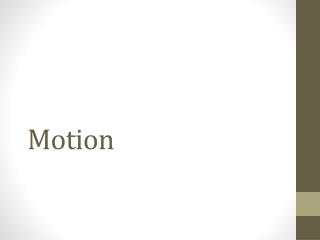# Motion - PowerPoint PPT PresentationDownload PresentationMotion

Presentation Description
Download Presentation## Motion

- - - - - - - - - - - - - - - - - - - - - - - - - - - E N D - - - - - - - - - - - - - - - - - - - - - - - - - - -
##### Presentation Transcript

1. Motion

2. Motion • An object is in motion if its distance from another object is changing

3. Reference Point • A place or object used for comparison to determine if something is in motion • An object is in motion if it changes position relative to a reference point Example If you are in a train, or car, why does the land look like it is moving but inside the train, or car, it doesn’t?

4. Speed vs. Velocity • Speed is the distance an object travels in a given amount of time. • Velocity is speed with a direction • The weather man likes to use velocity for wind.

5. Average Speed • Ex: a race in an amount of time. You don’t always run with the same amount of speed. • Instantaneous speed • The rate of speed at that exact time.

6. Graphing Motion • Distance vs. Time • Slope = rise/ run • Time = x axis = run • Distance = y axis = rise • Steepness = slope • Ex: 400 meters in 2 minutes • = 400m/ 2 minutes • = 200 meters per minute • Lab with marbles and lids. Page 318 • Books to elevate. • Three trials, with three levels.